# Anti-Lie triple system

(diff) ← Older revision | Latest revision (diff) | Newer revision → (diff)

A triple system is a vector spaceover a fieldtogether with a-trilinear mapping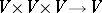. A triple systemsatisfying(a1)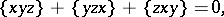(a2)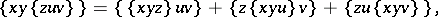(a3)

for all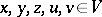, is called an anti-Lie triple system.

If instead of (a1) one has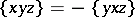, a Lie triple system is obtained.

Assume thatis an anti-Lie triple system and that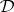is the Lie algebra of derivations ofcontaining the inner derivation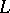defined by. Consider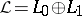with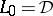and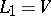, and with product given by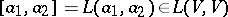,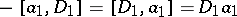,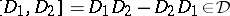for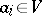,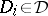(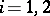). Then the definition of anti-Lie triple system implies that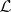is a Lie superalgebra (cf. also Lie algebra). Hence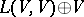is an ideal of the Lie superalgebra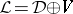. One denotesbyand calls it the standard embedding Lie superalgebra of. This concept is useful to obtain a construction of Lie superalgebras as well as a construction of Lie algebras from Lie triple systems.

How to Cite This Entry:
Anti-Lie triple system. Encyclopedia of Mathematics. URL: http://encyclopediaofmath.org/index.php?title=Anti-Lie_triple_system&oldid=16792
This article was adapted from an original article by Noriaki Kamiya (originator), which appeared in Encyclopedia of Mathematics - ISBN 1402006098. See original article